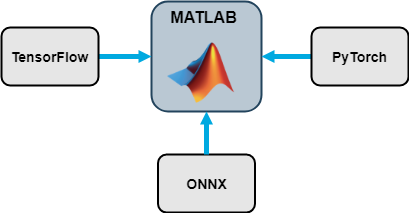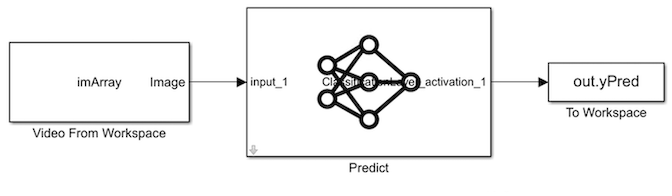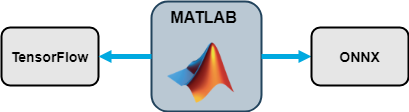## Interoperability Between Deep Learning Toolbox, TensorFlow, PyTorch, and ONNX

This topic provides an overview of the Deep Learning Toolbox™ import and export functions and describes common deep learning workflows that you can perform in MATLAB® with an imported network from TensorFlow™, PyTorch®, or ONNX™. For more information on how to overcome hurdles when you import networks, see Tips on Importing Models from TensorFlow, PyTorch, and ONNX.

Many pretrained networks are available in Deep Learning Toolbox. For more information, see Pretrained Deep Neural Networks. However, MATLAB does not stand alone in the deep learning ecosystem. Use the import and export functions to access models available in open-source repositories and collaborate with colleagues who work in other deep learning frameworks.

### Support Packages for Interoperability

You must have the relevant support packages to run the Deep Learning Toolbox import and export functions. If the support package is not installed, each function provides a download link to the corresponding support package in the Add-On Explorer. A recommended practice is to download the support package to the default location for the version of MATLAB you are running. You can also directly download the support packages from File Exchange.

This table lists the Deep Learning Toolbox support packages for import and export, the File Exchange links, and the functions each support package provides.

By using ONNX as an intermediate format, you can interoperate with other deep learning frameworks that support ONNX model export or import.

Note

The `importTensorFlowNetwork` and `importTensorFlowLayers` functions are recommended over the `importKerasNetwork` and `importKerasLayers` functions. For more details, see Recommended Functions to Import TensorFlow Models.

### Functions that Import Deep Learning Networks#### External Deep Learning Platforms and Import Functions

This table describes the deep learning platforms from which the Deep Learning Toolbox functions import models and which model formats they can import.

External Deep Learning PlatformModel FormatImport Model as NetworkImport Model as Layer Graph
TensorFlow 2`SavedModel` format`importTensorFlowNetwork``importTensorFlowLayers`
TensorFlow-KerasHDF5 format`importKerasNetwork``importKerasLayers`
PyTorchTraced model file with the `.pt` extension`importNetworkFromPyTorch`Not applicable
ONNXONNX model format`importONNXNetwork``importONNXLayers`

Each import and export function reference page contains a Limitations section which describes the supported versions of the external platform. For example, for the TensorFlow versions that the `importTensorFlowNetwork` function supports, see Limitations.

#### Objects Returned by Import Functions

This table describes the Deep Learning Toolbox network or layer graph that the import functions return when you import a pretrained model from TensorFlow, PyTorch, or ONNX.

Import FunctionDeep Learning Toolbox ObjectExamples
`importTensorFlowNetwork``DAGNetwork` or `dlnetwork`Import TensorFlow Network as DAGNetwork to Classify Image, Import TensorFlow Network as dlnetwork to Classify Image
`importTensorFlowLayers``LayerGraph`Import TensorFlow Network as Layer Graph Compatible with DAGNetwork, Import TensorFlow Network as Layer Graph Compatible with dlnetwork
`importKerasNetwork``DAGNetwork` or `SeriesNetwork`Import and Plot Keras Network
`importKerasLayers``LayerGraph` or `Layer` arrayImport Keras Network Layers and Train Network
`importNetworkFromPytorch`Uninitialized `dlnetwork`Import Network from PyTorch and Classify Image
`importONNXNetwork``DAGNetwork` or `dlnetwork`Import ONNX Network as DAGNetwork, Import ONNX Network with Autogenerated Custom Layers
`importONNXLayers``LayerGraph`Import ONNX Model as Layer Graph Compatible with DAGNetwork, Import ONNX Model as Layer Graph Compatible with dlnetwork
`importONNXFunction`Model function and `ONNXParameters` objectPredict Using Imported ONNX Function

After you import a network or layer graph, the returned object is ready for all the workflows that Deep Learning Toolbox supports.

#### Automatic Generation of Custom Layers

The `importTensorFlowNetwork`, `importTensorFlowLayers`, `importNetworkFromPyTorch`, `importONNXNetwork`, and `importONNXLayers` functions create automatically generated custom layers when you import a model with TensorFlow layers, PyTorch layers, or ONNX operators that the functions cannot convert to built-in MATLAB layers. The functions save the automatically generated custom layers to a package in the current folder. For more information, see Autogenerated Custom Layers.

### Visualize Imported Network

Use the `plot` function to plot a diagram of the imported network or layer graph. You cannot use the `plot` function with `dlnetwork` objects.

```net = importTensorFlowNetwork("digitsNet"); plot(net)```Use the `analyzeNetwork` function to visualize and understand the architecture of the imported network or layer graph, check that you have defined the architecture correctly, and detect problems before training. Problems that `analyzeNetwork` detects include missing or unconnected layers, incorrectly sized layer inputs, an incorrect number of layer inputs, and invalid graph structures.

```net = importTensorFlowNetwork("NASNetMobile", ... OutputLayerType="classification",Classes=ClassNames); analyzeNetwork(net)```### Predict with Imported Model

#### Preprocess Input Data

Preprocessing data is a common first step in the deep learning workflow to prepare data in a format that the network can accept. The input data size must match the network input size. If the sizes do not match, you must resize the input data. For an example, see Import ONNX Network as DAGNetwork. In some cases, the input data requires further processing, such as normalization. For an example, see Import ONNX Network with Autogenerated Custom Layers.

You must preprocess the input data in the same way as the training data. Often, open-source repositories provide information about the required input data preprocessing. To learn more about how to preprocess images and other types of data, see Preprocess Images for Deep Learning and Preprocess Data for Deep Neural Networks.

#### Import Model as `DAGNetwork` Object and Predict

Import a TensorFlow model in the `SavedModel` format. By default, `importTensorFlowNetwork` imports the network as a `DAGNetwork` object. Specify the output layer type for an image classification problem and the class names.

```net = importTensorFlowNetwork("NASNetMobile", ... OutputLayerType="classification",Classes=ClassNames);```

Predict the label of an image.

`label = classify(net,Im);`

#### Import Model as `dlnetwork` Object and Predict

You must first convert the input data to a `dlarray` object. For image input data, specify the data format as `"SSCB"`. For more information on how to specify the input data format, see Data Formats for Prediction with dlnetwork.

```dlIm = dlarray(Im,"SSCB"); ```

Import a TensorFlow model in the `SavedModel` format as a `dlnetwork` object. Specify the class names. A `dlnetwork` does not have output layers.

```net = importTensorFlowNetwork("NASNetMobile", ... Classes=ClassNames,TargetNetwork="dlnetwork"); ```

Predict the label of an image.

```prob = predict(net,dlIm); [~,label] = max(prob);```

Note

The `importNetworkFromPyTorch` function imports the network as an uninitialized `dlnetwork` object. Before prediction, add an input layer or initialize the imported network. For examples, see Import Network from PyTorch and Add Input Layer and Import Network from PyTorch and Initialize.

#### Compare Prediction Results

To check whether the TensorFlow or ONNX model matches the imported network, you can compare inference results by using real or randomized inputs to the network. For examples that show how to compare inference results, see Inference Comparison Between TensorFlow and Imported Networks for Image Classification and Inference Comparison Between ONNX and Imported Networks for Image Classification.

You can use the imported network with the Predict block of Deep Learning Toolbox to classify an image in Simulink®. The imported network can contain automatically generated custom layers. For an example, see Classify Images in Simulink with Imported TensorFlow Network.#### Predict on GPU

The import functions do not execute on a GPU. However, the functions import a pretrained neural network for deep learning as a `DAGNetwork` or `dlnetwork` object, which you can use on a GPU.

• If you import the network as a `DAGNetwork` object, you can make predictions with the imported network on a CPU or GPU by using `classify` or `predict`. Specify the hardware requirements using the `ExecutionEnvironment` name-value argument.

For networks with multiple outputs, use the `predict` function and specify the `ReturnCategorical` name-value argument as `true`.

• If you import the network as a `dlnetwork` object, you can make predictions with the imported network on a CPU or GPU by using `predict`. The `predict` function executes on the GPU if the input data or network parameters are stored on the GPU.

• If you use `minibatchqueue` to process and manage the mini-batches of input data, the `minibatchqueue` object converts the output to a GPU array by default if a GPU is available.

• Use `dlupdate` to convert the learnable parameters of a `dlnetwork` object to GPU arrays.

`net = dlupdate(@gpuArray,net)`

### Transfer Learning with Imported Network

Transfer learning is common in deep learning applications. You can use a pretrained network as a starting point to learn a new task. Fine-tuning a network with transfer learning is usually much faster and easier than training a network with randomly initialized weights from scratch. You can quickly transfer learned features to a new task using a smaller quantity of training data. This section describes how to import a convolutional model from TensorFlow for transfer learning.

Import the `NASNetMobile` model as a layer graph and display its final layers.

```lgraph = importTensorFlowLayers("NASNetMobile", ... OutputLayerType="classification"); lgraph.Layers(end-2:end)```
```ans = 3×1 Layer array with layers: 1 'predictions' Fully Connected 1000 fully connected layer 2 'predictions_softmax' Softmax softmax 3 'ClassificationLayer_predictions' Classification Output crossentropyex```

The last layer with learnable weights is a fully connected layer. Replace this fully connected layer with a new fully connected layer in which the number of outputs is equal to the number of classes in the new data set. To learn faster in the new layer than in the transferred layers, increase the learning rate factors of the layer.

```learnableLayer = lgraph.Layers(end-2); numClasses = numel(categories(imdsTrain.Labels)); newLearnableLayer = fullyConnectedLayer(numClasses, ... Name="new_fc", ... WeightLearnRateFactor=10, ... BiasLearnRateFactor=10); lgraph = replaceLayer(lgraph,learnableLayer.Name,newLearnableLayer);```

The classification layer specifies the output classes of the network. Replace the classification layer with a new one that does not have class labels. The `trainNetwork` function automatically sets the output classes of the layer at training time.

```classLayer = lgraph.Layers(end); newClassLayer = classificationLayer(Name="new_classoutput"); lgraph = replaceLayer(lgraph,classLayer.Name,newClassLayer);```

For an example that shows the complete transfer learning workflow, see Train Deep Learning Network to Classify New Images. For an example that shows how to train a network imported as a `dlnetwork` object to classify new images, see Train Network Imported from PyTorch to Classify New Images.

#### Train Network on GPU

You can train the imported network on a CPU or GPU by using `trainNetwork`. To specify training options, including options for the execution environment, use the `trainingOptions` function. Specify the hardware requirements using the `ExecutionEnvironment` name-value argument. For more information on how to accelerate training, see Scale Up Deep Learning in Parallel, on GPUs, and in the Cloud.

### Deploy Imported Network

#### Deploy Imported Network with MATLAB Coder or GPU Coder

You can use MATLAB Coder™ or GPU Coder™ together with Deep Learning Toolbox to generate MEX, standalone CPU, CUDA® MEX, or standalone CUDA code for an imported network. For more information, see Code Generation.

• Use MATLAB Coder with Deep Learning Toolbox to generate MEX or standalone CPU code that runs on desktop or embedded targets. You can deploy generated standalone code that uses the Intel® MKL-DNN library or the ARM® Compute library. Alternatively, you can generate generic C or C++ code that does not call third-party library functions. For more information, see Deep Learning with MATLAB Coder (MATLAB Coder).

• Use GPU Coder with Deep Learning Toolbox to generate CUDA MEX or standalone CUDA code that runs on desktop or embedded targets. You can deploy generated standalone CUDA code that uses the CUDA deep neural network library (cuDNN), the TensorRT™ high performance inference library, or the ARM Compute library for Mali GPU. For more information, see Deep Learning with GPU Coder (GPU Coder).

The import functions return the network as a `DAGNetwork` or `dlnetwork` object. Both of these objects support code generation. For more information on MATLAB Coder and GPU Coder support for Deep Learning Toolbox objects, see Supported Classes (MATLAB Coder) and Supported Classes (GPU Coder), respectively.

You can generate code for any imported network whose layers support code generation. For lists of the layers that support code generation with MATLAB Coder and GPU Coder, see Supported Layers (MATLAB Coder) and Supported Layers (GPU Coder), respectively. For more information on the code generation capabilities and limitations of each built-in MATLAB layer, see the Extended Capabilities section of the layer. For example, see the Code Generation and GPU Code Generation sections of `imageInputLayer`.

#### Deploy Imported Network with MATLAB Compiler

An imported network might include layers that MATLAB Coder does not support for deployment. In this case, you can deploy the imported network as a standalone application using MATLAB Compiler™. The standalone executable you create with MATLAB Compiler is independent of MATLAB; therefore, you can deploy it to users who do not have access to MATLAB.

You can deploy only the imported network using MATLAB Compiler, either programmatically by using the `mcc` (MATLAB Compiler) function or interactively by using the Application Compiler (MATLAB Compiler) app. For an example, see Deploy Imported TensorFlow Model with MATLAB Compiler.

Note

Automatically generated custom layers do not support code generation with MATLAB Coder, GPU Coder, or MATLAB Compiler.

### Functions that Export Networks and Layer Graphs

When you complete your deep learning workflows in MATLAB, you can share the deep learning network or layer graph with colleagues who work in different deep learning platforms. By entering one line of code, you can export the network.

`exportNetworkToTensorFlow(net,"myModel")`You can export networks and layer graphs to TensorFlow and ONNX by using the `exportNetworkToTensorFlow` and `exportONNXNetwork` functions. The functions can export `DAGNetwork`, `dlnetwork`, and `LayerGraph` objects.

• The `exportONNXNetwork` function exports to the ONNX model format.

• The `exportNetworkToTensorFlow` function saves the exported TensorFlow model in a regular Python® package. You can load the exported model and use it for prediction or training. You can also share the exported model by saving it to `SavedModel` or `HDF5` format. For more information on how to load the exported model and save it in a standard TensorFlow format, see Load Exported TensorFlow Model and Save Exported TensorFlow Model in Standard Format.